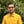Trusted answers to developer questions
Trusted Answers to Developer Questions

Related Tags

d
sin
math
communitycreator

# What is sin() in D?Harris Amjad

Grokking Modern System Design Interview for Engineers & Managers

Ace your System Design Interview and take your career to the next level. Learn to handle the design of applications like Netflix, Quora, Facebook, Uber, and many more in a 45-min interview. Learn the RESHADED framework for architecting web-scale applications by determining requirements, constraints, and assumptions before diving into a step-by-step design process.

The sin() function in D returns the sine of a number. To be more specific, sin() returns the sine of a number in radians.

The illustration below shows the mathematical representation of the sin() function.

$\sin (x)= \frac{\text {Length of the Opposite Side}}{ \text {Length of the Hypotenuse}}$

Notes:

• std.math is required for this function.
• The sin() function only works for right-angled triangles.

## Syntax

sin(number)
//number can be real, float, or double.


## Parameters

This function requires a number that represents an angle (in radians) as the parameter. In order to convert degrees to radians, use the following formula:

radians = degrees * ( PI / 180 )


## Return value

The sin() function returns the sine of the number (in radians) that is sent as a parameter.

## Code

The code below shows how to use the sin() function in D.

import core.stdc.stdio;import std.stdio;//header required for functionimport std.math;int main(){     //positive number in radians    writeln("The value of sin(2.3) ", sin(2.3));    // negative number in radians    writeln("The value of sin(-2.3) ", sin(-2.3));    //converting the degrees angle into radians and then applying sin()    // degrees = 90.0    // PI = 3.14159265    // result first converts degrees to radians then apply sin    double result=sin(90.0 * (PI / 180.0));    writeln("The value of sin(90.0 * (PI / 180.0)) ", result);    return 0;}
Code Sample

### Explanation

• Line 9: We declare a positive number in radians.
• Line 12: We declare a negative number in radians.
• Line 18: We convert the degrees into radians and then apply the sin() function. The variable result first converts degrees to radians and then applies sin().
• Line 19: We display the result.

RELATED TAGS

d
sin
math
communitycreator

CONTRIBUTORHarris Amjad

Grokking Modern System Design Interview for Engineers & Managers

Ace your System Design Interview and take your career to the next level. Learn to handle the design of applications like Netflix, Quora, Facebook, Uber, and many more in a 45-min interview. Learn the RESHADED framework for architecting web-scale applications by determining requirements, constraints, and assumptions before diving into a step-by-step design process.

Keep Exploring

Learn in-demand tech skills in half the time